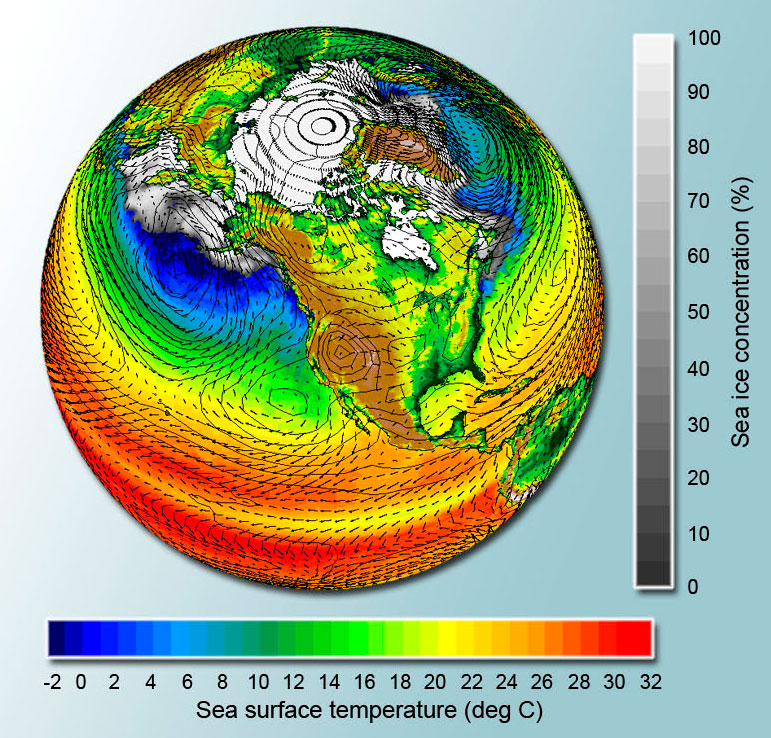# Why/What Mathematics for Engineers?

a critical analysis

Citation
, XML
Authors

# Abstract

Mathematics education for engineers is today the same as 50-100 years ago, while the computer has radically changed the mathematics of engineering practice . The consequences of this mismatch are serious and education reform is needed, and possible.

This is a continuation of the knols

We start from the following contradiction of engineering/science education and practice, today:

• Mathematics education for engineers/scientists is the same as 50-100 years ago.
• The computer has radically changed the mathematics of engineering/science practice.Engineering class 1941 using slide rule.             Engineering class 2010 using computer.

How can this be? Answer: Because mathematics education for engineers is offered by mathematics departments dominated by pure mathematicians following a pre-computer tradition rooted in 17-19th century mathematics. The result is:

• computational mathematics useful in engineering practice is not taught in basic engineering education
• only in masters/graduate programs in computational science and engineering for few students
• basic engineering/science education does not use the potential of computational mathematics
• students/teachers/society loose time, energy and money on inadequate useless mathematics
• students turn away from engineering/science education
• society lack competent engineers/scientists capable of attacking todays challenges with todays tools.

## What Can Be Done?

In principle, the solution is simple: Just replace traditional mathematics with computational mathematics in engineering/science education. This would lead from the present out-dated education dominated by
• not useful and not understandable classical analytical mathematics

to a modern education based on

• useful and understandable computational/analytical mathematics

which would enable engineering/science education and practice to function together.

Of course, usefulness helps understanding and vice versa, while non-usefulness implies not understandable and vice versa.
Computational mathematics adds a turbo to analytical mathematics in the form of the computer and can help to make the dreams come true of many generations of mathematicians, scientists and engineers of modeling,
understanding and controling physical processes for the benefit of people and society…
In practice, the solution is difficult because in computational mathematics the questions and answers are largely different form those of classical analytical mathematics of the 19th century. The difficulties are exposed in The School of Babel of Mathematics and Why School Mathematics?.
In short, mathematics education represents, like the Catholic Church, a very stable belief and tradition. We are told that in a world where everything seems to change, we need something which does not change, and this can be the eternal truths of the Catholic Church (which in fact also change, but slowly) and mathematics
(which does not change since 1+1 = 2 for ever, but which in fact is nothing but computational math…).
The very change of society and engineering/science practice, is thus used as a motivation to not also change mathematics education. This is like insisting on sending messages by smoke signals instead of using the mobile telephone. True, there are disadvantages of mobiles but a society based on smoke signals would not work well (like a society based on analytical 17th century mathematics):

A engineering mathematics education based on computational mathematics is available as the  Body and Soul Applied Mathematics Reform Program with a web-version to appear as Icarus Computational Calculus.
The harvest of computational mathematics is just starting: